SYLLABUS  Previous: 4.1 Plain vanilla stock  Up: 4.1 Plain vanilla stock  Next: 4.1.2 Parameters illustrated with

4.1.1 The European Black-Scholes model for dummies

[ SLIDE 2 shares - random walk - experiments || VIDEO modem - LAN - DSL ]

Remember how the price of an option has been calculated in sect.3.1: with a small twist, this will show you how sophisticated Monte-Carlo simulations are carried out in practice. Consider a European call giving its holder the right to buy a share for a strike of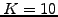EUR when the contract expires in 3 months or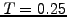year. For simplicity, assume that the terminal price of a share presently valued at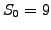EUR can take only one of two uncertain values with equal probabilities: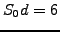andEUR. Using this probabilistic knowledge, it is easy to calculate the expected value at the expiry by weighting the terminal payoff (2.1.3#eq.1) from each realization with the probability factor 1/2: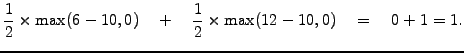Discounting back a quarter of year with a 3% continuous interest rate (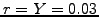in 1.3#eq.6), you immediately get the fair present value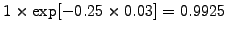EUR.

Even if the principle is correct, we showed in sect.3.2 that the forecasting values (3.2#eq.4) have to be carefully chosen to reproduce the market volatility, for example 40% (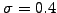): this yields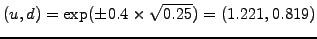and risk-neutral world probabilities (3.2#eq.3) where a movement up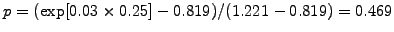is slightly less likely than a movement down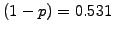. These parameters can again be used to calculate the expected payoff for different market values of the underlying share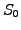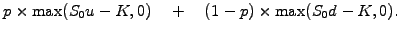After discounting by the same factor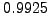, we get the present values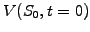shown in (4.1.1#tab.1) with the corresponding terminal payoff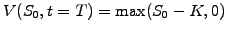.

Table 4.1.1#tab.1: Present and terminal value of a call obtained using a binomial step.7 8 9 10 11 12 130 0 0.46 1.03 1.6 2.17 3.07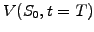0 0 0 0 1 2 3

Almost the same procedure is used in a Monte-Carlo calculation, except that for a higher accuracy, the lifetime of the option is divided into smaller steps: increments are accumulated to simulate possible realizations starting from the initial (spot) price of the underlying (log-normal walk with shares in sect.2.1.1) and set the drift equal to the spot rate (risk-neutral evolution observed for trees in sect.3.2). After each time step, the arithmetic average from all the possible terminal payoffs is used to estimate the mean price of the option on the expiring date and is discounted back in time to plot the fair value of an option having a lifetime equal to the run time. The VMARKET applet below shows an example with (Volatility=0.4, Drift=0.03), where new increments are generated every trading day (the duration of one step is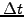=1/252=0.00397 year) and are accumulated to forecast two possible realizations of the underlying spot price (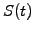, red dots) starting from an initial 10 EUR (coincides with the StrikePrice). After one step backward in time (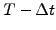or Time=0.003 displayed on the top of the applet measures the lifetime ranging from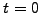to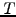), the fair value of the option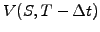is plotted (in black) together with the terminal payoff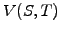(in grey). Running the simulation for 3 months (Time=0.25), the prices obtained using the Monte-Carlo method can be cross-checked with the value obtained from the binomial step (4.1.1#tab.1): they are quite different!

 Virtual market experiments: price of call option using Monte-Carlo Execute a few runs to verify that the same initial condition yield different solutions three months before the option expires-a financial non-sense! Increase the number of random samples of the underlying and convince yourself that the solution converges to a reasonable value above approximatively Walkers=1000. N.B.: select Monte-Carlo to avoid plotting the prices in red. Back to Walkers=1, explain the option payoff dynamics resulting from the movements of a single realization by reviewing all the arguments above.

The experiments show that the numerical accuracy of the Monte-Carlo calculation can be improved by increasing the number of realizations: the values obtained approach those given in (4.1.1#tab.1) without reproducing them exactly. The difference is particularly striking for low values of the underlying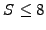, where the binomial step gives options that are worthless, while the Monte-Carlo method converges to small but finite values. As you may have guessed, also binomial trees are only an approximation of the true solution, with an accuracy that improves when the number of steps is increased-resulting in a larger number of forecasting prices. As a matter of fact, both methods converge to the same value in the limit of small time steps and a large number of realisations: this value is the same as the one that has first been obtained by Black & Scholes  by solving (3.4#eq.4).

Congratulations: you probably solved your first option pricing equation and hopefully even understood what you were doing! Of course, analytical minded persons may say that a formula is more general and provides a better understanding. In this syllabus, we argue the opposite: formulas, just like computers, are only tools to obtain solutions from a certain model of the reality. Analytical and computational tools are both perfectly adequate if they are used in a knowledgable manner: they are often favourably compared to ensure that the solution is not affected by different assumptions made during the derivation of the models.

Before tackling these issues, let us first develop an intuition for the financial parameters and study with experiments how they affect the option payoff before the expiry date.

SYLLABUS  Previous: 4.1 Plain vanilla stock  Up: 4.1 Plain vanilla stock  Next: 4.1.2 Parameters illustrated with